# TN006 Advanced Frequency Compensation Method for VF-TLP Measurement (up to 10 GHz)

### 1. Objective

The objective of this article is to demonstrate a frequency compensation technique for measuring the current and voltage of a device under test in a Very Fast Transmission Line Pulser (VF-TLP) test environment. The current measurement utilizes Non-Overlapping Time Domain Reflectometry, which is useful for On-Wafer testing because the measurement can be made with low profile small pitch probes, such as the Picoprobe Model 10. Further, to increase the bandwidth of the current measurement over common techniques, such as current transformers with 1GHz bandwidth, the method utilizes a resistive Pick-Off. The Pick-Off can be finely tuned to have as little insertion loss as possible, thereby enhancing the bandwidth. Although this method can also yield a DUT voltage measurement, the result suffers from numerical errors for low ohmic devices. A separate, direct measurement is presented that will demonstrate an extremely accurate voltage measurement that also utilizes frequency compensation.

### 2. Measurement Setup

Very Fast Transmission Line Pulse or VF-TLP testing is a difficult measurement to perform well. This is a high bandwidth measurement and all connections must be completed as well as possible to maintain the characteristic impedance of the system (typically 50 ). This translates into ensuring that any probe connections are well matched, and any connectors/adaptors are of the highest possible quality. Further, due to how Non-Overlapping TDR measurements are implemented, there are great benefits from using low loss cables, which are very expensive.

The overall measurement setup is depicted in Figure 1, where it can be seen to the far right hand of the figure that the system can accommodate a variety of DUT connection methods. In particular, the pulse is applied to the DUT by the Pulse In connection, and a direct voltage measurement is taken at the Vm connection. A true Kelvin measurement can be done using two probes, providing a good On-Wafer measurement solution. Also seen in the figure are an ES 621 Transmission Line Pulser, a Pick-Off tee, a leakage control module (A621-LTKSEM), a Source Meter Unit (SMU), and an Oscilloscope. Encircled numbers denote where frequency domain measurement points are located for the Non-Overlapping TDR current measurement. Under normal pulsing conditions, the leakage control module connections are in the Normally Closed (NC) positions. And during leakage current measurement conditions, the module is in the Normally Open (NO) positions. The NO position connects the DUT to the SMU, and disconnects the voltage measurement resistor Rv. A photograph of the setup is shown in Figure 2.Figure 1 ESDEMC VF-TLP Measurement SetupFigure 2 ESDEMC VF-TLP Delay Line Cable Arrangement

#### 2.1 Current Measurement

A simplified figure highlighting the Non-Overlapping TDR connections of the measurement is shown in Figure 3. From the left, a TLP connects to Port 1 of the cabling layout. The V+ indicates the positive outgoing wave from the TLP. Next in line is the resistive Pick-Off, where the incident or (V+M) measurement is taken as V+ propagates toward the DUT. After reaching the DUT, a reflected wave (V-L) propagates back toward the Pick Off to be measured as V-M. The cable between the Pick-Off and the DUT is labeled the Delay Line. This is because the length of this cable must be long enough to delay the reflected wave from reaching the Pick-Off before the incident wave has fully passed. This can be guaranteed if the Delay Line is at least two times longer than the pulse width. The Delay Line length and the need for low loss cables typically results in pulse widths no longer than 10 ns for this measurement method.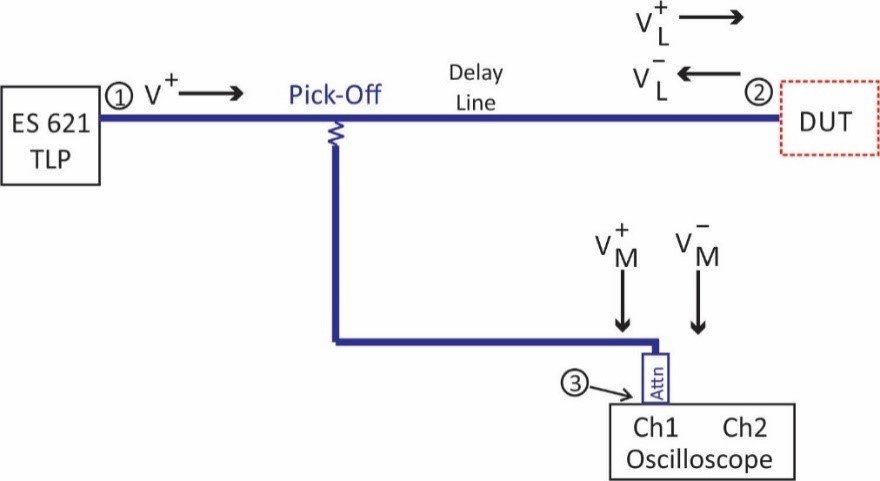Figure 3 ESDEMC VF-TLP Non-Overlapping TDR connections

The encircled numbers (○1, ○2, and ○3) in Figure 3 represent where the frequency compensation measurements must be made. In particular, S21, S31, and S32 must be measured and saved for future use for each measurement setup. If any cables or attenuators, or the Pick-Off are changed, then the S-parameters must be re-measured. The leakage control module must be included in the measurement, and put in the normally closed state. The magnitude of the three S-parameters for a typical setup are shown in Figure 4. It would be desirable for the insertion loss of the cabling and Pick-Off to be 0 dB across all frequencies, allowing the full TLP output pulse to be applied to the DUT. However, real low loss cables can have a few dB of attenuation at 10’s of GHz. For the measured setup the increased loss is most likely from the Pick-Off, and the SMA connectors between the ports for connecting the leakage relay and the DUT. Another item to note is that the S21 measurement is relatively flat.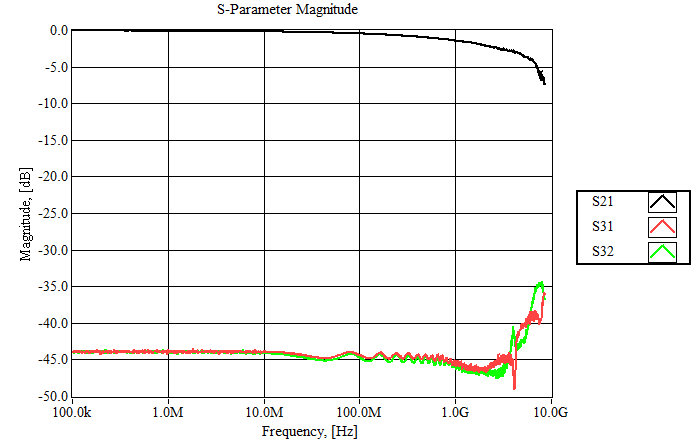Figure 4 Magnitude of S-parameter measurements for the Non-Overlapping TDR setup

The other two S-parameter measurements are very similar, and this is to be expected because other than S32 having the longer Delay Line in the path, the connections are identical. The ripple in the magnitude measurements are due to reflections in the connections. The ripple is approximately 1 dB to 1 GHz. How these measured S-parameters are used will be presented in Section 3.

#### 2.2 Voltage Measurement

As stated earlier, the Non-Overlapping TDR measurement does yield a DUT voltage measurement. However, the measurement suffers from numerical errors for low ohmic devices. This is due to the Transmission Line theory math used in the processing of the captured data, and will be explained in greater detail in Section 3. A good measurement of the DUT voltage can be achieved by a separate, direct measurement, and a simplified connection diagram for this is shown in Figure 5.
This measurement is typically very accurate with just using the probe ratio and attenuator value to calculate the DUT voltage. However, with VF-TLP type measurements having higher bandwidth restrictions, a more accurate measurement can be achieved by using frequency compensation. Like Non-Overlapping TDR, the cable loss, attenuator value and connection quality can all be represented and compensated for by capturing the S21 of the measurement system. The magnitude of the S21 measurement taken for the system is shown in Figure 6.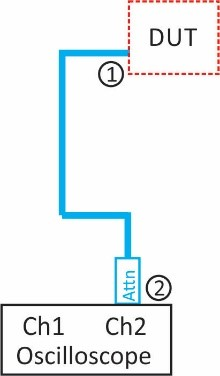Figure 5. VF-TLP Direct Voltage Measurement Setup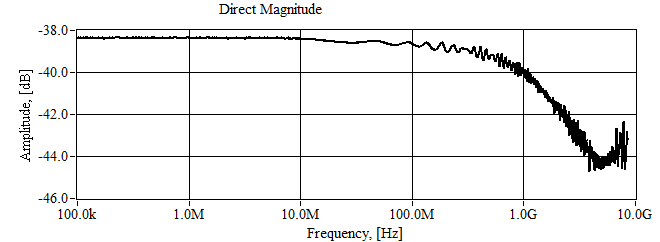Figure 6 Magnitude of S-parameter measurements for the Voltage Measurement

For reasons that will be explained in the next section, the probe connection for the measurement in Figure 6 is the PCB, or upper right hand sub-figure, in Figure 1. The measurement is relatively flat to 1 GHz.

### 3. Frequency Compensation

Processing of the captured data requires loading of the S-parameter data into the TLP measurement control code. This is easily accomplished using the Touchstone file format that all network analyzers support. The core of the processing in this method is done in the frequency domain, which means that the measured time waveforms must be transformed to the frequency domain. Since it is nearly impossible to ensure that the time record length is identical to the measured S-parameters, interpolation must be done to obtain equal length data sets.

A flow diagram for the Non-Overlapping TDR measurement for IDUT is shown in Figure 7. At the upper left hand of the figure is shown a captured Non-Overlapping TDR waveform plot. In the plot, the left hand pulse is the Pick-Off measured incident pulse (V+M), and the right hand pulse is the Pick-Off measured reflected pulse (V-M). The next step in processing gets the data prepared for transforming to the frequency domain. In this step the incident and reflected pulses are separated, Windowed, Filtered, and Interpolated. In particular, the pulses are separated at the Cut Point, which is arbitrary, and merely needs to be in between the two pulses. If the Delay Line is altered or the pulse width changed, the Cut Point should be verified to be in an adequate position. Windowing is required to force to zero the beginning and ending of the time record to ensure that the captured data appear periodic in regard to the FFT. If this is not done, the FFT result will suffer from spectral leakage. Next, if desired, the data can be filtered. In the results presented herein there was no filtering of the data. Finally, the time and frequency domain data are interpolated.

The next step in the flow diagram is to transform the time data by Fast Fourier Transform (FFT). There is other mathematical manipulation not shown herein, but the math is illustrated in the next step of the flow diagram. This manipulation translates the two Pick-Off measurements (V+M and V-M) to the Load/DUT. In particular, the measured incident wave (V+M) must be translated back to the TLP (divide by S31), and then translated to the Load (multiplication by S21), yielding V+L. Since the measured reflected wave (V-M) only traverses between the Load and the measurement port, it only requires translation back to the load by dividing by S32, yielding V-L.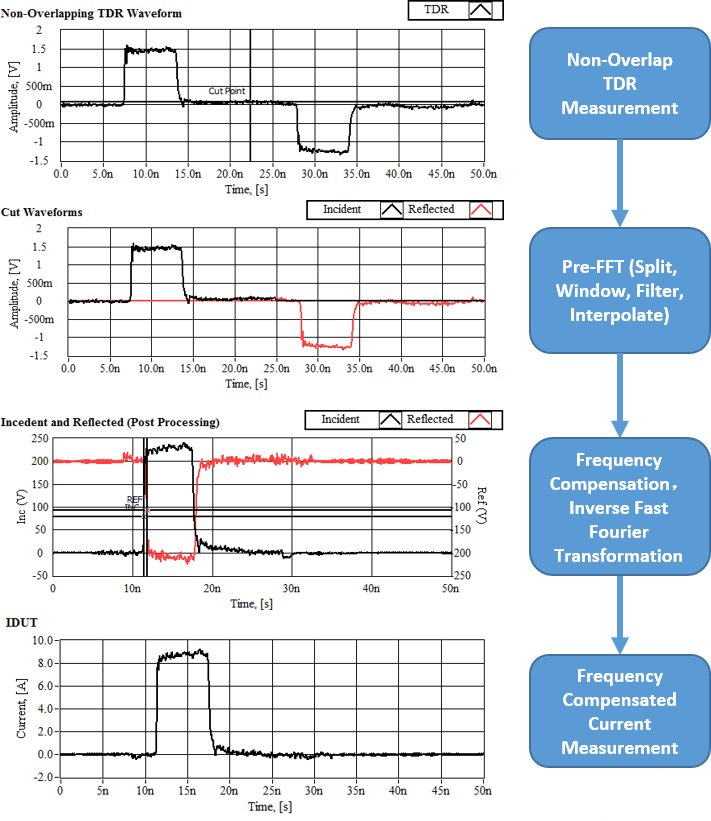Figure 7. ESDEMC VF-TLP Non-Overlapping TDR Processing Flow Diagram

Time domain representations of the incident and reflected waves at the Load are then obtained by Inverse-FFT (IFFT). The two waveforms are shown in the plot to the left of the IFFT flow diagram step, and a zoomed in version is shown in Figure 8. Notice that there is a time difference between these two pulses. The S-parameter measurements should have taken care of this time difference, and given the exact same connections they would have. However, since SMA adaptors must be used to connect to the various different ports, all the measurements could not be placed at the calibration plane. Fortunately, the difference is short (typically pico-seconds) which can be accounted for by subtracting the time from the reflected pulse.Figure 8. V+L and V-L Waveforms (Zoom).

Finally, the Load current or IDUT can then be calculated as shown in the last step of the flow diagram. Notice for low ohmic devices the resulting pulses are comparable in magnitude and in opposite directions. This combination is good for determining the current as the result is the addition of two numbers of comparable size. This is then divided by the characteristic impedance to obtain the current. As stated previously, this is not a good scenario for measuring the DUT voltage. Considering the same pulses, of comparable magnitude and opposite directions, the result for the voltage is the subtraction of two similar numbers, and potential for greater error. This method works great for voltage measurements of high ohmic devices, but for similar reasons the current measurement would suffer.

Processing for the direct voltage measurement is very similar to the current measurement, and the flow diagram is shown in Figure 9. The data is Windowed, Filtered (if desired), and Interpolated before being transformed by FFT. The frequency domain manipulation merely translates the measurement recorded at the oscilloscope back to the Load by dividing by S21 of the measurement path. Shown in the upper left, and bottom left hand of Figure 9 are the raw and resulting voltage at the DUT waveforms, respectively. Notice how the compensation removed the excessive ringing on the voltage measurement introduced by the probe.Figure 9. ESDEMC VF-TLP Voltage Measurement Flow Diagram

### 4. Measurement

The goal of the method described above is to obtain the best possible, high bandwidth measurement possible, and the method utilizes Frequency compensation to try and achieve this goal. To demonstrate the ability of the technique a comparison of two different voltage measurements, recorded simultaneously, was performed. To do this a 1  resistor was mounted onto a PCB along with a measuring resistor (Rv), as demonstrated in the upper right hand of Figure 1. There is one exception to the connections of Figure 1 in that the addition of a Thru port that can be fed directly to the oscilloscope has been added. A schematic and photograph of the PCB are shown in Figure 10. The DUT is connected from Pulse In to ground, making the effective DUT a parallel combination of the 1  resistor with the oscilloscope input impedance (1 Ohm||50 Ohm). The Thru side measurement is directly connected to the oscilloscope channel 3 with a 30 dB / 18 GHz attenuator. The Thru measurement is compensated for by value of the attenuator only.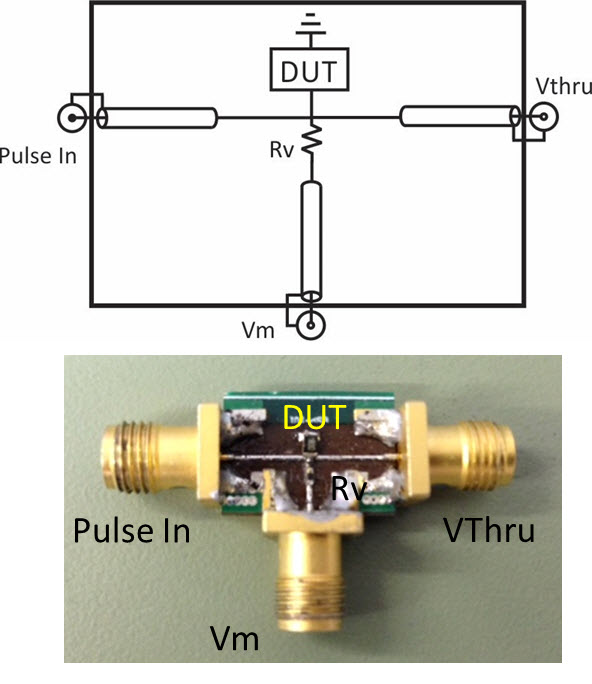Figure 10. Photo of VF-TLP DUT test PCB

To truly test and understand the ability of the system, a brief introduction into the pulse shape characteristics that will be used to pulse the DUT are presented. First, the ES 621 TLP can produce a very clean and fast rising pulse, as depicted in Figure 11. The measurement was performed with a Tektronix MSO 72304DX (23 GHz/100 GSa/s) oscilloscope, a short 18 GHz cable and attenuator. As can be seen in the lower left hand of the figure, the average rise time measurement is approximately 60 ps, and the average pulse width measurement is approximately 1.04 ns. Further, the overshoot is small and settles quickly.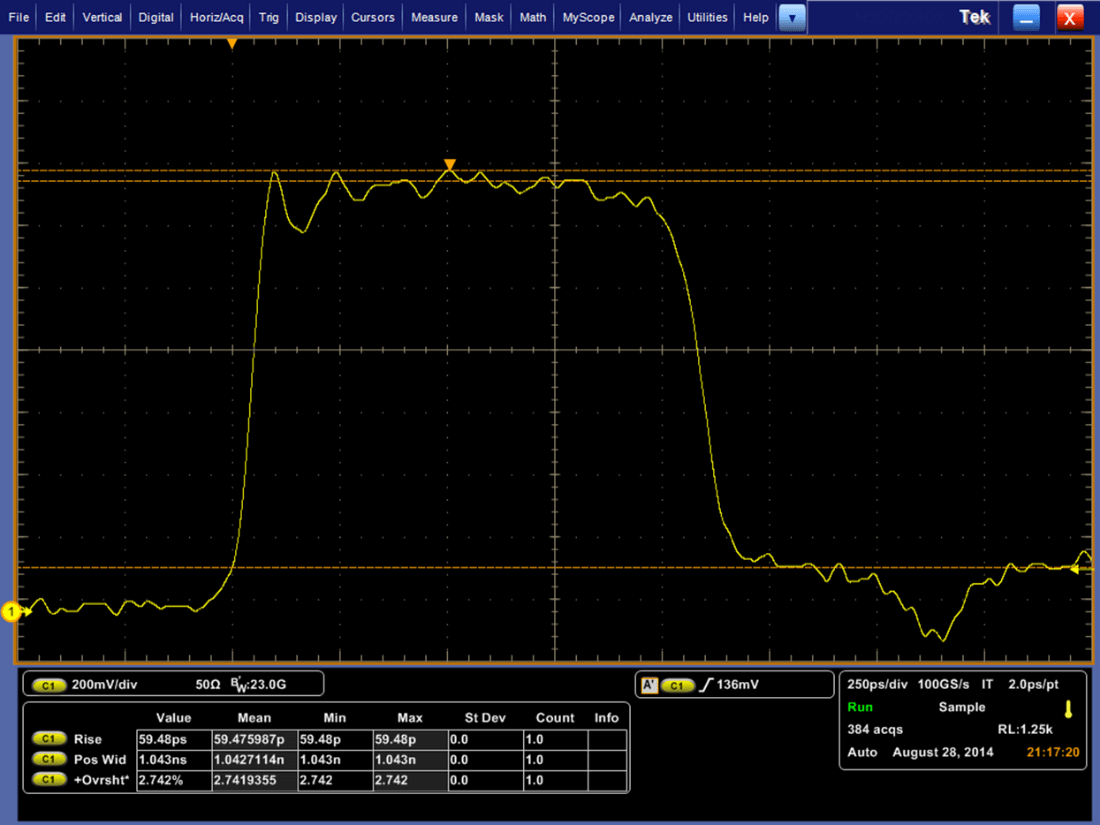Figure 11. ES 621 Vf-TLP output, 60 ps rise time, 1ns pulse, with 23 GHz Oscilloscope, Measured in ESDEMC Headquarter Rolla, MO, USA 2014/08/28

Shown in Figure 12 are results for a current measurement. The Black trace is the raw result. By raw, it is meant that the final measured current value is determined by aligning the incident and reflected waveforms in time, scaling them by the Pick-Off probe value and attenuator in the measurement path, and then using the equation at the bottom of the flow diagram in Figure 7. The Red trace is the same measurement, but it is the result after the frequency compensation method described above. As can be seen the two measurements are in good agreement in regard to general shape and amplitude. But, the frequency compensated measurement removes artifacts introduced by the measurement probe, such as the high frequency ripple at the beginning of the current pulse.Figure 12 ESDEMC VF-TLP Raw and Frequency Compensated Current Measurement

Shown in Figure 13 are results for a voltage measurement. The Black trace is the raw direct voltage measurement, scaled by the probe factor and attenuator value only. The Red trace is the same measurement, but it is the result after the frequency compensation method described above. The Blue trace is VThru and is the result of the direct voltage measurement and a 30 dB / 18 GHz attenuator as the only compensation. As can be seen, the Red and Blue traces match very well, supporting the frequency compensation technique does an extremely good job in obtaining the voltage measurement.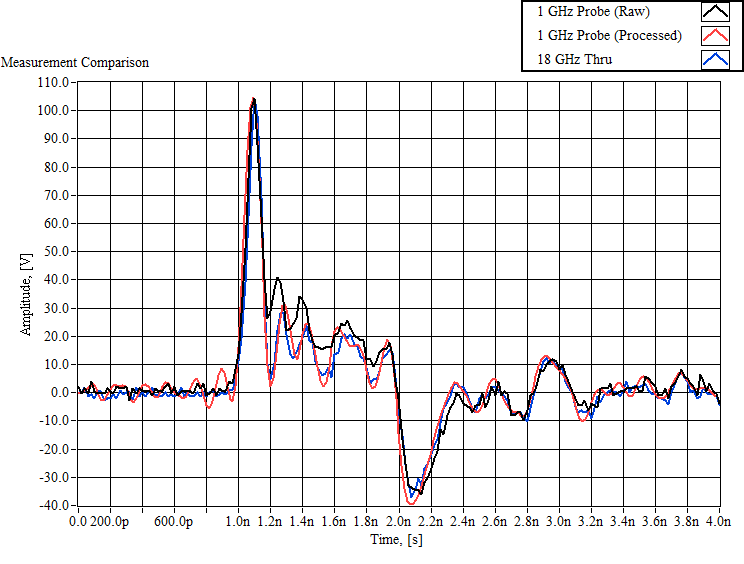Figure 13. Raw, Frequency Compensated, and Thru Voltage Measurement

### 5. Conclusions

The objective of this article was to demonstrate a frequency compensation technique for measuring the current and voltage of a device under test in a Very Fast Transmission Line Pulser (VF-TLP) test environment. It was shown that by incorporating the S-parameter measurements of the test setup into the processing routine that an extremely good voltage measurement could be obtained. In particular, a high bandwidth direct voltage measurement was used to verify the VF-TLP voltage measurement. Similarly, the compensation method was used to obtain the device current from the Non-Overlapping TDR measurement.ESDEMC VF-TLP Measurement of 60ps rise-time 1ns VF-TLP Pulse

#### Appendix: Measurement Equipments

Tektronix MSO 72304DX, 23 GHz/100 GSa/s, Oscilloscope
ESDEMC ES621 Transmission Line Pulser System
ESDEMC A621-LTKSEM Leakage Control Module
ESDEMC FS312 VF-TLP Pick-Off
Suhner Sucoflex 100 high performance microwave cable
Micro-Coax UTiFlex Ultra low loss microwave cable
Mini-Circuits SMSM Cable
Gore-Tex UltraLow Loss Coax Cable